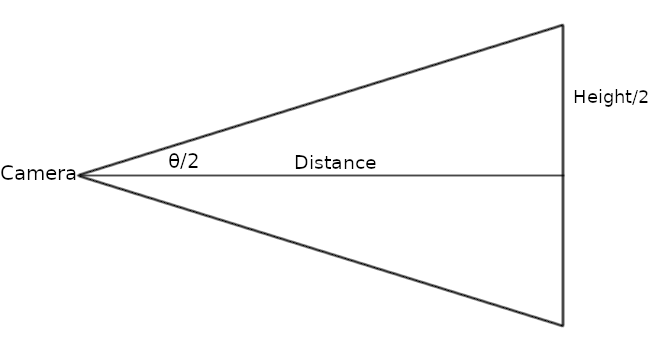# Enable parallel projection without resetting the camera

Hi, Support!

When I press P to enable parallel projection, the camera is “restarted”. Is this a bug? Must I reimplement the transformations to overcome this problem or is there a simpler solution?

Thanks for any help!

parallel_projection_bug.py
``````import vtk

def keyboard_callback(object, event):
if object.GetKeyCode() == 'p':
camera = renderer.GetActiveCamera()
flag = camera.GetParallelProjection()
camera.SetParallelProjection(not flag)

source = vtk.vtkSphereSource()

mapper = vtk.vtkPolyDataMapper()
mapper.SetInputConnection(source.GetOutputPort())

actor = vtk.vtkActor()
actor.SetMapper(mapper)
actor.GetProperty().EdgeVisibilityOn()

renderer = vtk.vtkRenderer()
renderer.AddActor(actor)
renderer.SetBackground(.3, .3, .3)

window = vtk.vtkRenderWindow()
window.AddRenderer(renderer)
window.SetSize(800, 600)

interactor = vtk.vtkRenderWindowInteractor()
interactor.AddObserver('KeyPressEvent', keyboard_callback)
interactor.SetRenderWindow(window)
interactor.SetInteractorStyle(vtk.vtkInteractorStyleRubberBandPick())
interactor.Start()
``````

Probably it is not a bug. These two projections are completely different, so you cannot keep camera parameters when switching. If for your application you don’t find VTK’s behavior optimal then you can compute the new camera parameters before switching and set them in the camera after switching projection mode.

@lassoan Thank you for answering me. I will check these parameters.

The parallel vs. perspective projection are different but not completely different. There are lots of parameters that they have in common:

• Camera Position
• Camera FocalPoint
• ClippingRange

The big difference is that parallel projection uses “ParallelScale”, but perspective uses “ViewAngle”. But even these parameters are closely related, because they are just two different ways of describing the height of the view (ParallelScale describes the height as a length, while ViewAngle describes the height as an angle).

And, of course, lengths and angles are related to each other through trigonometry, so ParallelScale and ViewAngle are in fact mathematically related:

``````ParallelScale = 2*(Distance*tan(0.5*ViewAngle))
``````

In this equation the “Distance” is the distance from the camera to the focal point (which is usually the same as the distance from the camera to the object of interest).

So, given a perspective view it’s generally possible to compute a good parallel view and vice-versa. But VTK does not do this.

1 Like

What I meant is that parallel and perspective projections are fundamentally different, so it is not possible to compute parameters that results in generally equivalent views.

I think VTK’s behavior is reasonable. I would not expect vtkCamera to change any other of its parameters when I call SetParallelProjectionOn/Off. Instead, when the application switches between projection modes then it can compute the parameters that are equivalent for the particular use case (or call generic conversion methods available in vtkRenderer: `ResetCamera(bounds)`, `ResetCameraScreenSpace(bounds)`).

However, the original poster specifically asked for methods that do not involve resetting the camera. My reading of the question was, is there a way to switch between perspective and parallel projection that keeps an (approximately) spherical object the same size within the view?

My reading of the question was, is there a way to switch between perspective and parallel projection that keeps an (approximately) spherical object the same size within the view?

@dgobbi Yes, I would like to do exactly that, as shown below (fake image).
@lassoan Sorry for not being clear on my question.

@lassoan and @dgobbi Thank you both very much for the explanations and suggestions. They will help me to try to overcome this problem.

### To: @dgobbi

Dear Gobbi,

The equation you provided is producing different results than VTK. I found another equation in the VTK code that solved the problem:

``````ParallelScale = Distance * sin(0.5*ViewAngle)
``````

Thank you very much for your help!

### To: @lassoan

Dear Lasso,

I solved the problem by setting the position and the parallel projection scale of the active camera.

@dgobbi is right! There is a geometric relationship between parallel and perspective projections. I used this relationship to enable/disable the parallel projection of a same camera without producing the “reset camera” effect.

You’re right! It’s not a bug. However, I see this problem as an unwanted effect that could be solved within the VTK.

Thank you for your help!

### Solution

parallel_projection_bug_solved.py
``````import vtk
import math

def keyboard_callback(caller, event):
if caller.GetKeyCode() == 'p':
camera = renderer.GetActiveCamera()
angle = camera.GetViewAngle()
angle = math.radians(angle)
if camera.GetParallelProjection():
point = camera.GetFocalPoint()
direction = camera.GetDirectionOfProjection()
scale = camera.GetParallelScale()
distance = scale / math.sin(.5 * angle)
x = point - distance * direction
y = point - distance * direction
z = point - distance * direction
camera.SetPosition(x, y, z)
camera.ParallelProjectionOff()
renderer.ResetCameraClippingRange()
else:
distance = camera.GetDistance()
scale = distance * math.sin(.5 * angle)
camera.SetParallelScale(scale)
camera.ParallelProjectionOn()
window.Render()

source = vtk.vtkSphereSource()

mapper = vtk.vtkPolyDataMapper()
mapper.SetInputConnection(source.GetOutputPort())

actor = vtk.vtkActor()
actor.SetMapper(mapper)
actor.GetProperty().EdgeVisibilityOn()

renderer = vtk.vtkRenderer()
renderer.AddActor(actor)
renderer.SetBackground(.3, .3, .3)
renderer.GetActiveCamera().ParallelProjectionOn()
renderer.ResetCamera()

window = vtk.vtkRenderWindow()
window.AddRenderer(renderer)
window.SetSize(800, 600)

interactor = vtk.vtkRenderWindowInteractor()
interactor.AddObserver('KeyPressEvent', keyboard_callback)
interactor.SetRenderWindow(window)
interactor.SetInteractorStyle(vtk.vtkInteractorStyleRubberBandPick())
interactor.Start()
``````

Hi Rodrigo,

I might have been mistaken about the factor of two, but not about using “tan()”. If sin() seems to work, that’s only because sin() and tan() give similar results for small angles.

Using sin() would only be correct if “Distance” was measured along the hypotenuse. But it’s not. This can be shown with a very simple diagram.Hi @dgobbi,

You’re right again! I didn’t try to understand, but VTK uses `sin` instead of `tan`. I had to use `sin` to produce the same result as VTK. Thank you for your attention!

Source: vtkRenderer.cxx (line 1162)